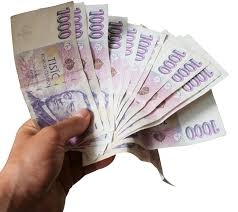# Precisely 7199

If Peter had 700 CZK more, he would have the same as Jirka. Zdenek has a third of what Peter precisely. Together they have 7000 CZK. How much does everyone have?

j =  3400
p =  2700
z =  900

### Step-by-step explanation:

p+700=j
z = p/3
p+j+z = 7000

j-p = 700
p-3z = 0
j+p+z = 7000

Row 3 - Row 1 → Row 3
j-p = 700
p-3z = 0
2p+z = 6300

Pivot: Row 2 ↔ Row 3
j-p = 700
2p+z = 6300
p-3z = 0

Row 3 - 1/2 · Row 2 → Row 3
j-p = 700
2p+z = 6300
-3.5z = -3150

z = -3150/-3.5 = 900
p = 6300-z/2 = 6300-900/2 = 2700
j = 700+p = 700+2700 = 3400

j = 3400
p = 2700
z = 900

Our linear equations calculator calculates it.Did you find an error or inaccuracy? Feel free to write us. Thank you!

Tips for related online calculators
Need help calculating sum, simplifying, or multiplying fractions? Try our fraction calculator.
Do you have a system of equations and looking for calculator system of linear equations?